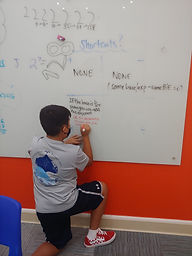## Ms. Mari

### Target 1​

###### Lesson Type:

Review

Number Operation

:

Computation

Simplify exponential expressions.

###### 1:

Identify that when multiplying a whole number by a power of ten, the product will have an increased number of zeros, that has a direct relationship to the exponent.

6th

###### Vocabulary:

BASE, EXPONENT

Activities:

- Used a card game to practice evaluating exponential expressions and comparing the results.

- For each round, each student drew two cards -- the first card was the base, the second was the exponent.

- The student with the higher value claimed the cards from that round.

- Discussed whether having a higher base or a higher exponent gave a greater result.

- Powers of 10 were reviewed in this exercise as well.### Home Exploration

###### Guiding Questions:## Absent Students:

Mikayla

### Target 2

:

###### 1:

Solve multi-step exponential expressions involving addition and subtraction.

###### 2:

Determine that there are no “short-cuts” for the addition and subtraction of exponential expressions (no matter what the bases or exponents are).

###### 3:

Determine the exponential rules or laws that apply to multiplication and division.

8th

###### Vocabulary:

BASE, EXPONENT, POWER

Activities:

- Simplified expressions involving four operations (+, -, x, /) and exponential expressions. (Completed WB p. 55, 56, 58, 60)

- Determined whether there were any "shortcuts" for simplifying the expressions.

- Discovered that for addition/subtraction, there were none, but for multiplication, there were two "shortcuts":

1. Same base: add the exponents
2. Same exponents: multiply bases, then apply the exponent### Home Exploration

###### Guiding Questions:### Target 3

:

###### Vocabulary:

Activities:### Home Exploration